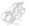# GTU Question papers Engineering Graphics

GUJARAT TECHNOLOGICAL UNIVERSITY

BE SEM- I / II Winter Examination-Dec.-2011

Subject code: 110013

Subject Name: Engineering Graphics

Instructions:

1. Attempt any five questions.
2. Make suitable assumptions wherever necessary.
3. Figures to the right indicate full marks.
4. Lines, dimensions etc. should be as per BIS SP-46.
5. Retain all construction line.

Q.1 (a) Explain the difference between 1st angle and IIIrd angle orthographic projection. 03 (b) Figure No.1 shows the Pictorial view of an object. Draw the following views 11 using first angle projection method (a) Sectional Front elevation looking from direction X, take section along A-A (b) Top view (c) Side view from left. Show all dimensions.

Q.2 (a) Figure No.2 shows the four bar chain mechanism O1ABO2 and the dimensions of 07 are as below :

O1A = O2B = 1125 mm                        connecting link AB = 375 mm

Draw the locus of midpoint M of AB, considering O1A as driving link.

(b) A circle of 50 mm diameter rolls along the circumference of another circle of 150 07 mm diameter from outside. Draw the path of a point P on the circumference of the rolling circle for one complete revolution and name the curve.

Q.3 (a) A straight AB has its end A 10 mm above HP and end B 50 mm in front of the 07 V.P. Draw the projections of line AB, if it is inclined to H.P. by 30° and to V.P. by 450 and it is 50 mm long.

(b) ABCD is a rhombus of diagonals AC = 110 mm and BD =70 mm. Its corner A is 07 in the H.P. and the plane is inclined to H.P. such that the plan appears to be a square. The plan of diagonal AC makes an angle of 200 to the V.P. Draw the projections of the plane and find its inclination with H.P.

Q.4 (a) The top view of 75 mm lone line AB measures 65 mm, while the length of its 07 front view is 50 mm. Its one end A is in the H.P. and 12 mm in front of the V.P. Draw the projections of line AB and its inclinations with the H.P. and the V.P.

(b) The top plan of a pair of equal legs AB and AC of compass appears as an 07 isosceles triangle having base 50 mm and vertex angle at 450 .Actual lengths of compass legs AB and AC are 120 mm. Assume points B and C on H.P. and line connecting B and C is perpendicular to V.P.. Draw the projections and find ( I ) the actual angle between two legs ( ii ) the height of point above HP and ( iii ) angle of plane, containing compass, makes with H.P.

Q.5 (a) Engineering graphics is a language of all persons involved in engineering 03 activities. Discuss the statement.

(b) A cone, base 75 mm diameter and axis 80 mm long is resting on its base on the 11 H.P. It is cut by a section plane perpendicular to the V.P., inclined at 450 to the H.P. and cutting the axis at a 35 mm from the apex. Draw front view, sectional top view and true shape of the section.

Q.6 (a) Write short note on BIS SP-46 engineering drawing standard.                                                     03

(b) A pentagonal pyramid, side of base 40 mm and height 80 mm, is resting on its 11 base with one of the edges of the base away from V.P. is parallel to V.P. It is cut by an AIP bisecting the axis, the distance of the section plane from the apex being 20 mm. Draw the elevation and sectional plan of pyramid and draw the true shape of the section. Also find the inclination of the section plane with the H.P.

Q.7 (a) Define development of surfaces and state its applications in engineering field. 03 (b) A hollow square pyramid, side of base 45 mm and height 65 mm, is resting on 11 H.P. on its base with all sides of base equally inclined to V.P. A square hole of size 20 mm is drilled through the pyramid. Sides of the square hole are equally inclined to H.P. Axis of the square hole and pyramid intersects at right angle 20 mm above the base of the pyramid. Axis of the hole is perpendicular to V.P. Draw the plan, elevation and development of the lateral surfaces of the pyramid.Fig No.2 Question 2 (a)